ODEcalc 6.1 ODEcalc (tm): Ordinary Differential Equation (ODE) Calculator! Solves ODEs Freeware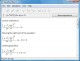Equation Wizard 1.21 Automatically solves algebraic equations of any order written in any form SharewarePDF Equation 0.7 PDF Equation gives you quick access to LaTeX's typesetting power in almost every Mac OS X application. FreewareEquation Service 1.0 Equation Service is a program that uses pdflatex to produce small PDF files containing equations and other text. FreewareChemical Equation Expert 2.12a Chemical Equation Expert is an integrated tool for chemistry professionals Freeware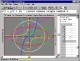Graphmatica 2.4 powerful, easy-to-use, equation plotter with numerical and calculus features Shareware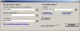PEEI 4.0.0.1 Solver of systems of partial differential equations. FreewareBerkeley Madonna 8.3.15 Berkeley Madonna is arguably the fastest, most convenient, general purpose differential equation solver available today. Shareware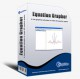Equation Grapher 2.2 Equation grapher is a free graphing calculator to graph equations. Freeware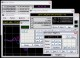Kalkulator 2.41 Advanced Sci/Eng calculator, from simple expressions to differential equations Shareware